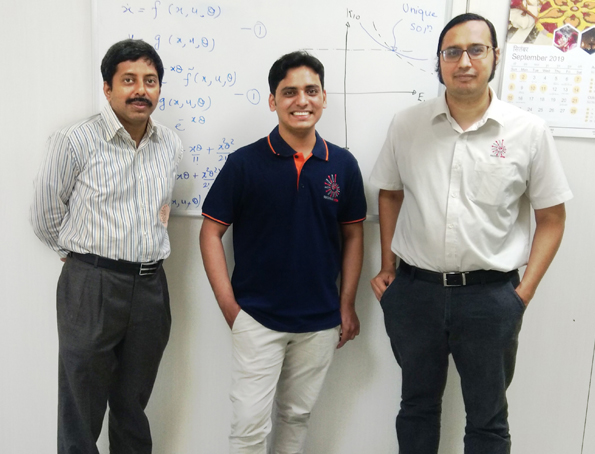# IIT-Madras team suggests a method to solve Mathematical Models with “non-rational” functionsMathematics has been considered a nightmare by many during their school days. While subjects like physics, biology and chemistry seemed to have practical applications, students often found mathematics dry and were confused about the utility of mathematics in everyday life, beyond basic algebra.

However, mathematicians firmly believe that mathematics is quite exciting and finds applications in almost every field of knowledge, whether physical sciences, social sciences or economics. Mathematical modelling, a process that uses mathematics to represent the behavior of any real-world phenomenon, allows one to analyze and make predictions about the system when certain changes are made in the system. The system here can be understood as the brain in neurosciences, the water cycle in ecology, a reaction in chemistry and biology, the stock market in economics or a rice cooker in a kitchen.

A mathematical model is essential for various industries and governance as it allows one to predict future conditions, which enable individuals to make a well-informed decision. For example, these models can be used for predicting the spread of infections in various regions of the body, based on the various variables such as population density, temperature and so on, which influence the spread. Similarly, modelling can help in predicting the traffic at a given route based on various parameters. In business, it can help to optimize production as per the sale needs for maximizing profit.

“The mathematical models are developed from data obtained by performing a systematic design of experiments. Before we spend resources and time to generate data for modelling, it is important to answer the following question: Is it possible to identify the unknown parameters in the given model structure uniquely? This question can be answered by
a set of techniques, called a priori parameter identifiability”, says Dr. Nirav Bhatt, Assistant Professor at IIT Madras and author of the study.

Rational numbers are those numbers which can be written as a ratio of two integers, whereas non-rational numbers such as Pi is non-terminating and non-repeating. Likewise, rational functions can be expressed as ratio of polynomial functions. While modelling can help us predict the outcomes of a system when the mathematical equation contains rational functions, the analysis of systems that have nonrational functions is difficult.

“Parameter identifiability methods in the literature can be applied to model structures having rational functions. However, in certain applications in biological reactions and chemistry, these model structures are non-rational functions of parameters. In this case, the approaches in the literature cannot be applied to answer a priori parameter identifiability. Hence, there is a need to develop an approach to answer a priori parameter identifiability for the nonrational model structures,” said Prof. Sridharakumar Narasimhan Professor at IIT Madras and author of the study.Prof. Sridharakumar Narasimhan, Rishabh Jain and Dr. Nirav Bhatt (Left to Right) who developed a method to solve mathematical models with non-rational functions.

A team of researchers from IIT Madras comprising of Rishabh Jain, Sridharakumar Narasimhan, and Nirav Bhatt has come up with a method to solve this problem and have published the method in the journal Automatica. The team has successfully developed a method for predicting the models with non-rational functions using two approximation methods (Pade and power series) and differential algebra. Further , the researchers showed that in certain systems, if the approximation of a certain order is used and the resulting system is identifiable, then higher-order approximations will also result in identifiable systems. The researchers demonstrated the utility of the proposed method in predicting outcomes of chemical reactions which are generally operating in varying temperature conditions.

Although the research has opened the avenues in the application of mathematical models, researchers feel that the method has high computational cost, i.e. long computation time, high memory and disk usage, among others,. which may restrict its applicability for complex systems. In future, the researchers intend to decrease the computational cost of the method so that it can be applied to large and complex reaction systems arising in biology and chemistry.

“Having a method for testing a priori identifiability of systems with non-rational functions significantly expands the class of systems on which such identifiability analysis can be carried out. This has practical implications in system identification / parameter estimation problems since models of many engineering systems such as those encountered in reacting systems involve non-rational functions. Being able to verify a priori identifiability of parameters will give confidence to the engineer/analyst in performing carefully designed experiments for actually estimating the parameters,” says Prof. Mani Bhushan, Professor at IIT Bombay, who is an expert in the field, but not associated with the study.